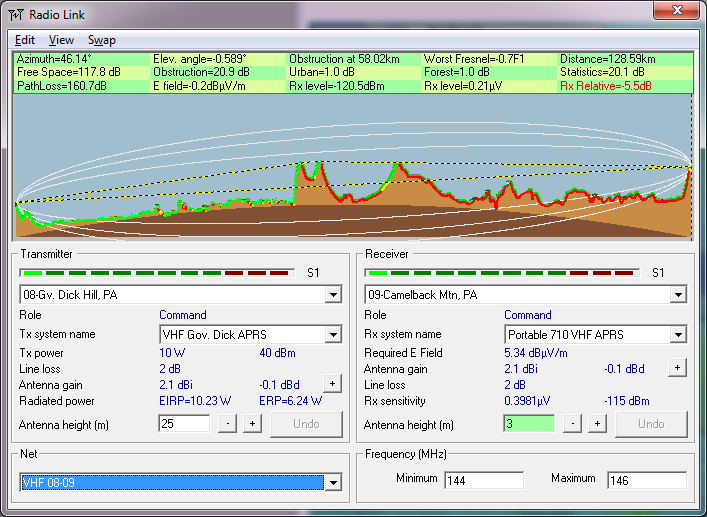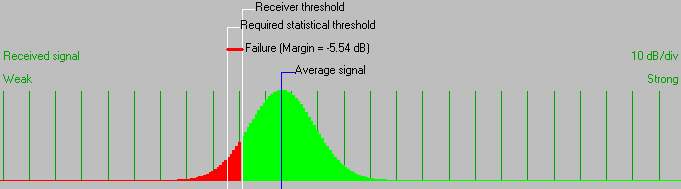• Distance between 08-Gv. Dick Hill, PA and 09-Camelback Mtn, PA is 128.6 km (79.9 miles)
• True North Azimuth = 46.14°, Magnetic North Azimuth = 57.55°, Elevation angle = -0.5891°
• Terrain elevation variation is 523.4 m
• Propagation mode is scatter, double horizon, 0.7F1 at 58.0km
• Average frequency is 145.000 MHz
• Free Space = 117.8 dB, Obstruction = 20.9 dB, Urban = 1.0 dB, Forest = 1.0 dB, Statistics = 20.1 dB
• Total propagation loss is 160.7 dB (interference mode (optimistic))
• System gain from 08-Gv. Dick Hill, PA to 09-Camelback Mtn, PA is 155.2 dB
• System gain from 09-Camelback Mtn, PA to 08-Gv. Dick Hill, PA is 155.2 dB
• Worst reception is 5.5 dB below the required signal to meet 95.000% of situations